Data Structures and Algorithms with Object-Oriented Design Patterns in C## Projects

1. Enhance the functionality of the RPN calculator given in Programin the following ways:
1. Use double-precision, floating-point arithmetic.
2. Provide the complete repertoire of basic operators: +, -,, and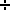.
3. Add an exponentiation operator and a unary negation operator.
4. Add a clear method that empties the operand stack and a print method that prints out the contents of the operand stack.
2. Modify Programso that it accepts expressions written in prefix (Polish) notation. Hint: See Exercise.
3. Write a program to convert a postfix expression into an infix expression using a stack. One way to do this is to modify the RPN calculator program given in Programto use a stack of infix expressions. A binary operator should pop two strings from the stack and then push a string which is formed by concatenating the operator and its operands in the correct order. For example, suppose the operator is ``*'' and the two strings popped from the stack are "(b+c)" and "a". Then the result that gets pushed onto the stack is the string "a*(b+c)".
4.   Devise a scheme using a stack to convert an infix expression to a postfix expression. Hint: In a postfix expression operators appear after their operands whereas in an infix expression they appear between their operands. Process the symbols in the prefix expression one-by-one. Output operands immediately, but save the operators in a stack until they are needed. Pay special attention to the precedence of the operators.
5. Modify your solution to Projectso that it immediately evaluates the infix expression. That is, create an InfixCalculator method in the style of Program.
6. Consider a string of characters, S, comprised only of the characters `(`, `)`, `[`, `]`, , and . We say that S is balanced if it has one of the following forms:
•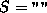, i.e., S is the string of length zero,
•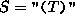,
•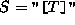,
•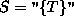,
•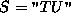where both T and U are balanced strings, In other words, for every left parenthesis, bracket or brace, there is a corresponding right parenthesis, bracket or brace. For example, "{()[()]}" is balanced, but "([)]" is not. Write a program that uses a stack of characters to test whether a given string is balanced.
7. Design and implement a MultipleStack class which provides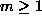stacks in a single container. The declaration of the class should look something like this:
```public class MultipleStack : Container
{
public MultipleStack(int numberOfStacks);
public void Push(object object, int whichStack);
public object Pop(int whichStack);
// ...
}```
• The constructor takes a single integer argument that specifies the number of stacks in the container.
• The Push method takes two arguments. The first gives the object to be pushed and the second specifies the stack on which to push it.
• The Pop method takes a single integer argument which specifies the stack to pop.
Choose one of the following implementation approaches:
1. Keep all the stack elements in a single array.
2. Use an array of Stack objects.
3. Use a linked list of Stack objects.
8. Design and implement a class called DequeAsDoublyLinkedList that implements the Deque interface using a doubly-linked list. Select one of the approaches shown in Figure.
9. In Section, the DequeAsArray class extends the QueueAsArray class. Redesign the DequeAsArray and QueueAsArray components of the class hierarchy making DequeAsArray the base class and deriving QueueAsArray from it.Figure: Expression tree for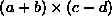.

10. Devise an approach for evaluating an arithmetic expression using a queue (rather than a stack). Hint: Transform the expression into a tree as shown in Figureand then do a breadth-first traversal of the tree in reverse (see Exercise). For example, the expressionbecomes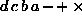. Evaluate the resulting sequence from left to right using a queue in the same way that a postfix expression is evaluated using a stack.Copyright © 2001 by Bruno R. Preiss, P.Eng. All rights reserved.